# How does a 7 segment display work | Arduino with 7 segment display | Step by Step instructions

## How does a 7 segment display work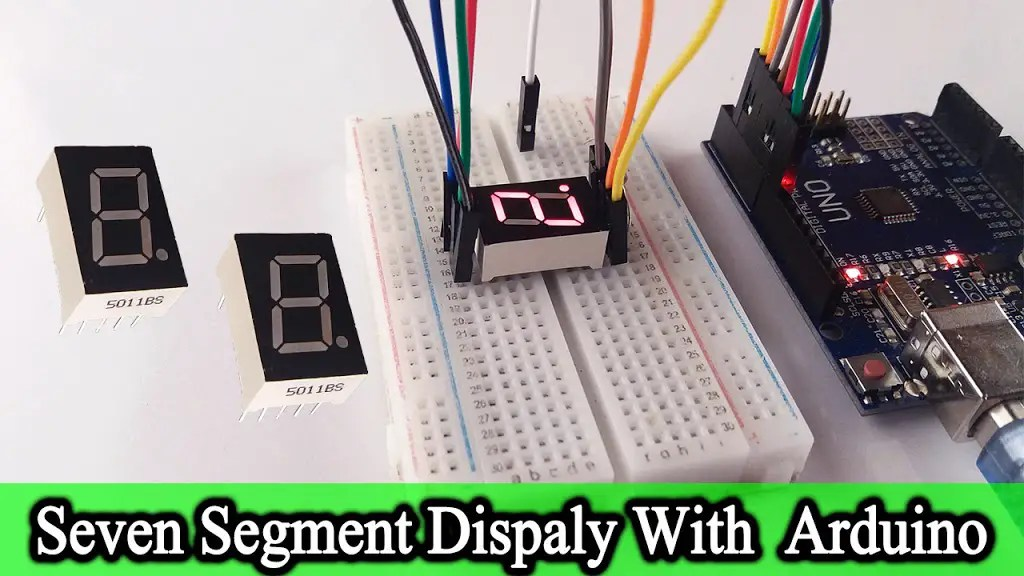Hello, welcome back. This tutorial includes, what is the seven-segment display and how does a 7 segment display work with Arduino. Also, these displays are called Seven segment displays because they are made up of seven parts. We can see this display in devices like alarm clocks, toys, counting systems, scoreboards. We can use this display to display characters from 0 to 9 and from A to Z. This tutorial explains step by step the functionality of this seven-segment display and how to use it with Arduino. I also believe that this knowledge will be more useful for your projects.

### What is a seven-segment display?

This display is called Seven segment display as it is made up of seven parts. It also has seven LEDs for the seven parts and one LED bulb for the decimal point. In terms of LED color, we can see these in red, blue, and orange in the market. Also, the LEDs in these seven parts are labeled a to g. Also known as the decimal point DP.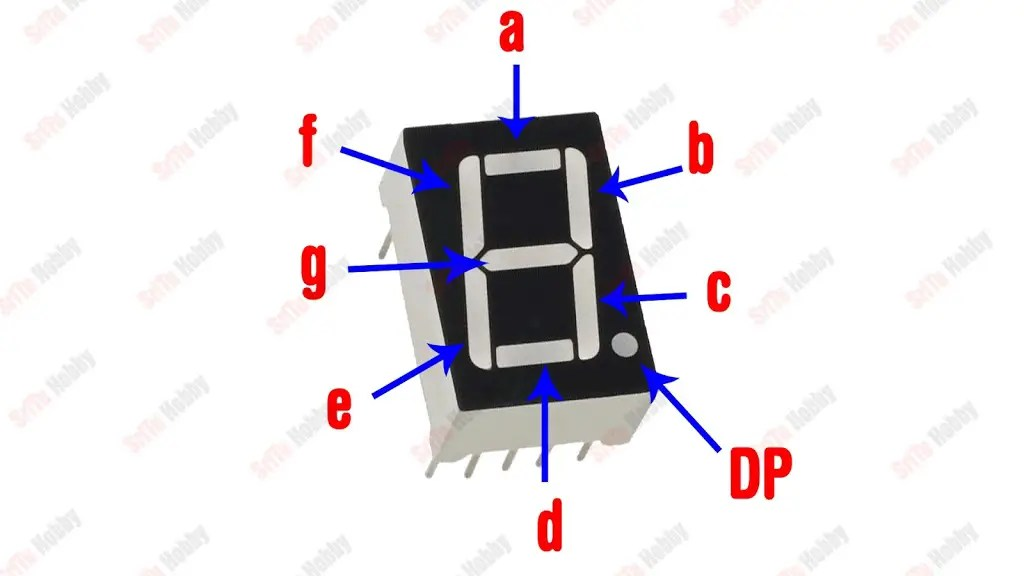Each of these LEDs has two pins. One of these pins is considered to be the common pin and all eight LEDs combine to form a common pin. We can light these ones by one through the other eight PINs. According to the common pin, there are two types of these displays.

#### 1.Common cathode Display

Here the common terminal is taken as the cathode. That is, the negative PINs of all eight LEDs are connected together. A negative voltage must be applied to this PIN. Also, by applying a separate positive voltage to the other eight terminals, we can turn LEDs on by one.#### 2.Common anode display

Here the positive pins of all eight LEDs are connected together. A positive voltage must be supplied to the common pin. Also, the parts can be lit one by one by supplying negative voltages to the other eight pins.### PIN structure of this DisplayOK, let’s learn how to use this display with Arduino step by step. To do this, the required components are given below.

### Step 1

Firstly, identify these components.

#### Arduino UNO board#### Seven segment display (Common anode display)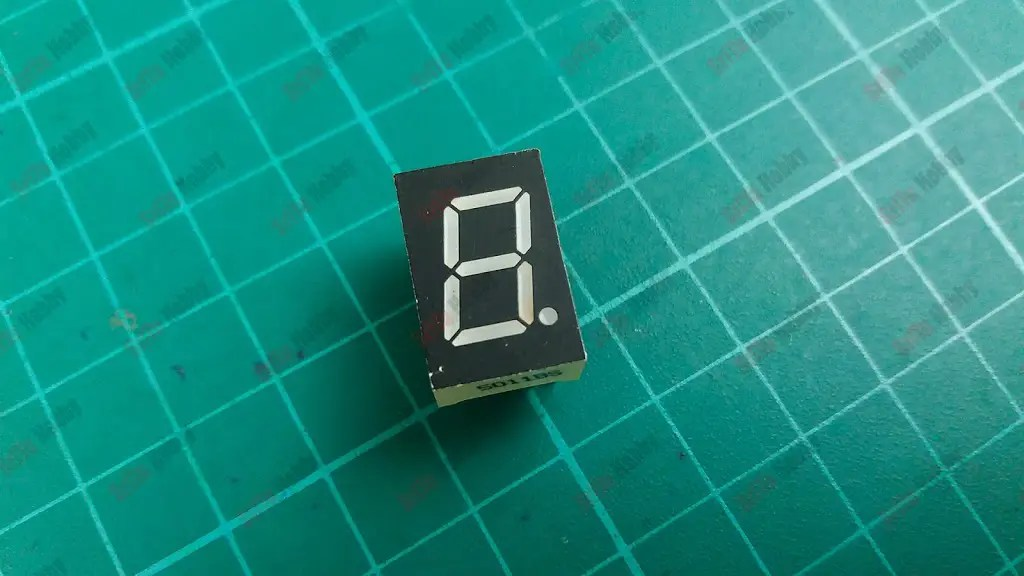#### Jumper wires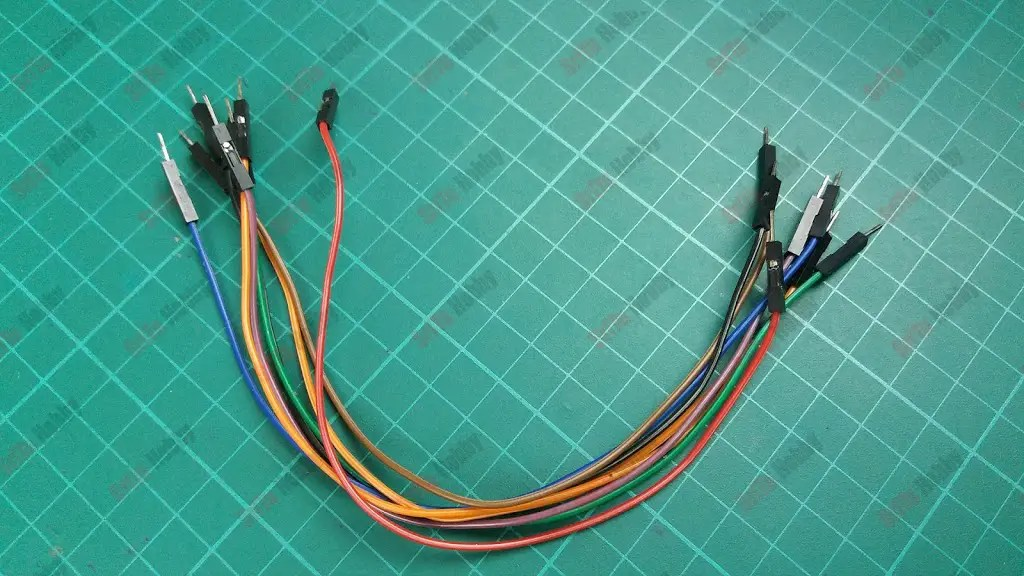### Step 2

Secondly, connect these components. For that, use the circuit diagram below. Also, the common anode display is used for this tutorial.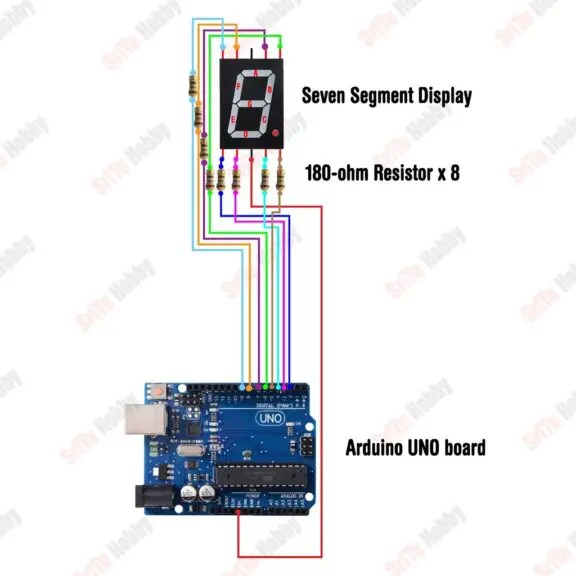### Step 3

Thirdly, let’s creates the program. It is as follows.
The complete program of this project – Download
``````/*How to use a seven-segment LED display.
created by the SriTu Tech team.
Read the code below and use it for any of your creations.
http://srituhobby.com
*/
byte pin[] = {2, 3, 4, 5, 6, 7, 8, 9};//arduino pin array

int number = {//number array
{1, 1, 0, 0, 0, 1, 1, 1},//1
{0, 0, 1, 0, 0, 0, 1, 0},//2
{1, 0, 0, 0, 0, 0, 1, 0},//3
{1, 1, 0, 0, 0, 1, 0, 0},//4
{1, 0, 0, 0, 1, 0, 0, 0},//5
{0, 0, 0, 0, 1, 0, 0, 0},//6
{1, 1, 0, 0, 0, 0, 0, 1},//7
{0, 0, 0, 0, 0, 0, 0, 0},//8
{1, 1, 0, 0, 0, 0, 0, 0},//9
};

void setup() {
for (byte a = 0; a < 8; a++) {
pinMode(pin[a], OUTPUT);//define output pins
}
}

void loop() {
for (int a = 0; a < 9; a++) {
for (int b = 0; b < 8; b++) {
digitalWrite(pin[b], number[a][b]);//display numbers
}
delay(500);//delay
}
}``````

#### Code explanation

First, an array is created for the segment display pins.
byte pin[] = {2, 3, 4, 5, 6, 7, 8, 9};//arduino pin array

Next, an array is created to put the numbers between 1 and 9.
int number = {//number array
{1, 1, 0, 0, 0, 1, 1, 1},//1
{0, 0, 1, 0, 0, 0, 1, 0},//2
{1, 0, 0, 0, 0, 0, 1, 0},//3
{1, 1, 0, 0, 0, 1, 0, 0},//4
{1, 0, 0, 0, 1, 0, 0, 0},//5
{0, 0, 0, 0, 1, 0, 0, 0},//6
{1, 1, 0, 0, 0, 0, 0, 1},//7
{0, 0, 0, 0, 0, 0, 0, 0},//8
{1, 1, 0, 0, 0, 0, 0, 0},//9
};
In the setup function, the display pins are set as the output pins.
void setup() {
for (byte a = 0; a < 8; a++) {
pinMode(pin[a], OUTPUT);//define output pins
}
}

In the loop function, the numbers from 1 to 9 are printed on the display.
void loop() {
for (int a = 0; a < 9; a++) {
for (int b = 0; b < 8; b++) {
digitalWrite(pin[b], number[a][b]);//display numbers
}
delay(500);//delay
}
}

### Step 4

OK, now select board and port, Afterward, upload this code.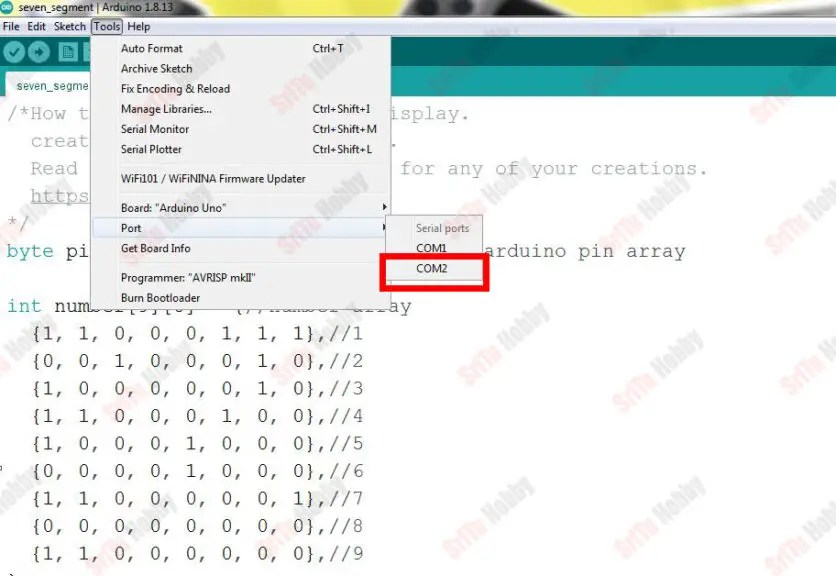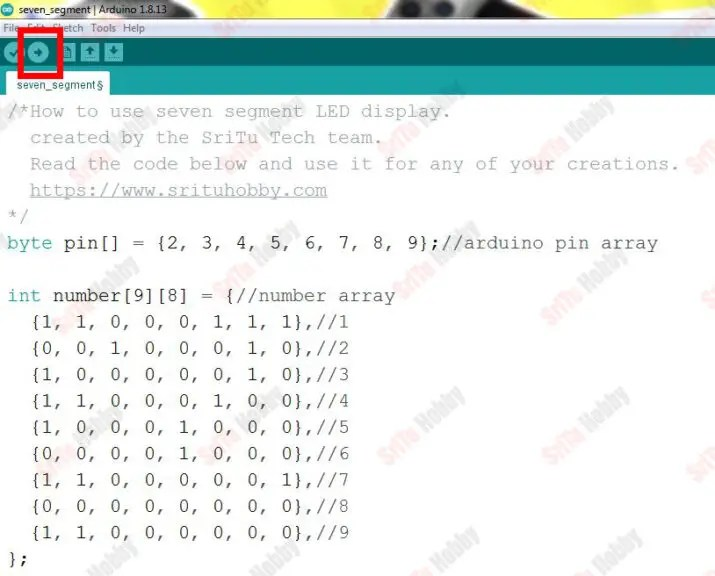OK, enjoy this project. The full video guide is given below. So, we will meet in the next tutorial.

How does a 7 segment display work | Arduino with 7 segment display | Step by Step instructions